## 算法策略 - 递归（Recursion）_玉树临风你卓哥的博客-程序员宅基地

• 递归：函数（方法）直接或间接调用自身。是一种常见的编程技巧
``````int sum(int n) {

if (n <= 1) return n;
return n + sum(n - 1);
}
``````
``````void a(int v) {

if (v < 0) return;
b(--v);
}
void b(int v) {

a(--v);
}
``````

## 递归现象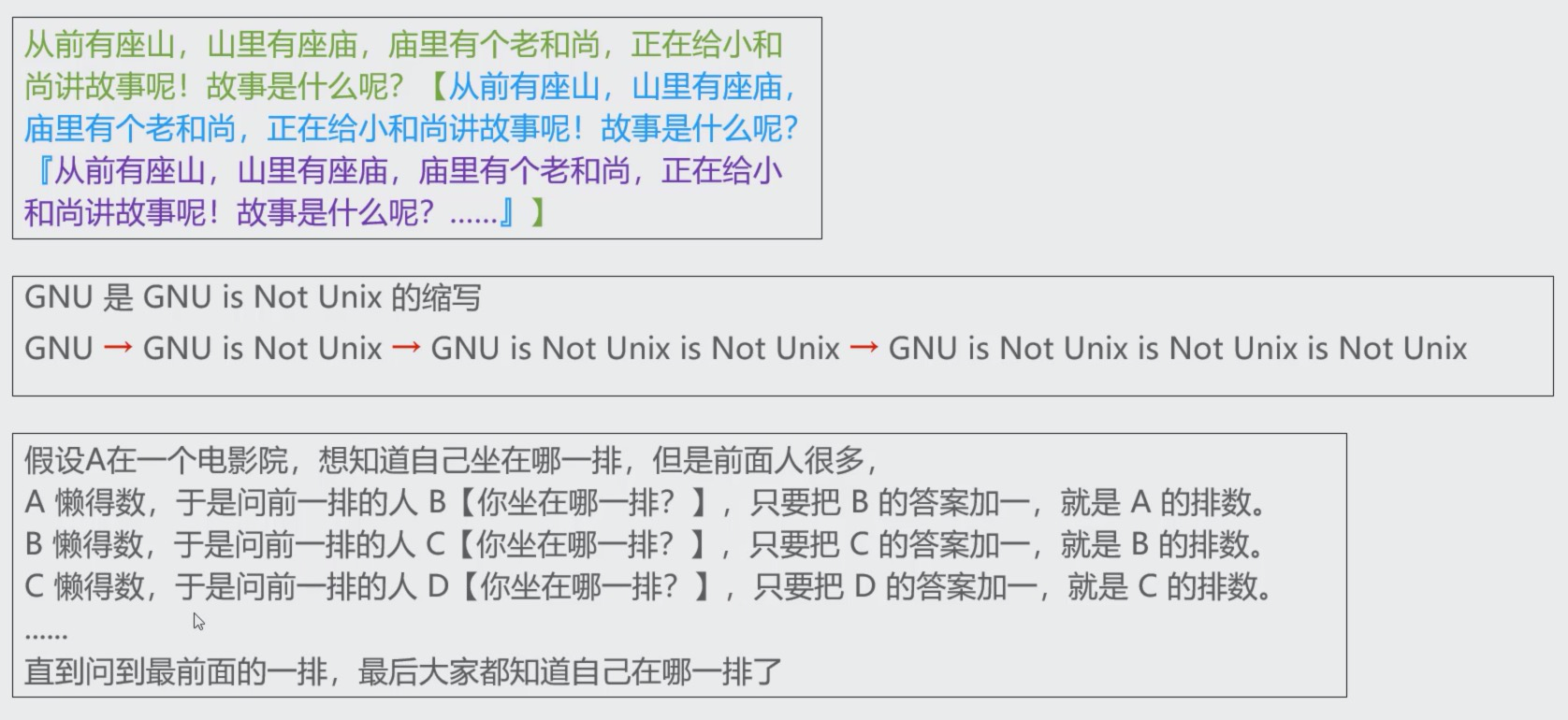## 函数的调用过程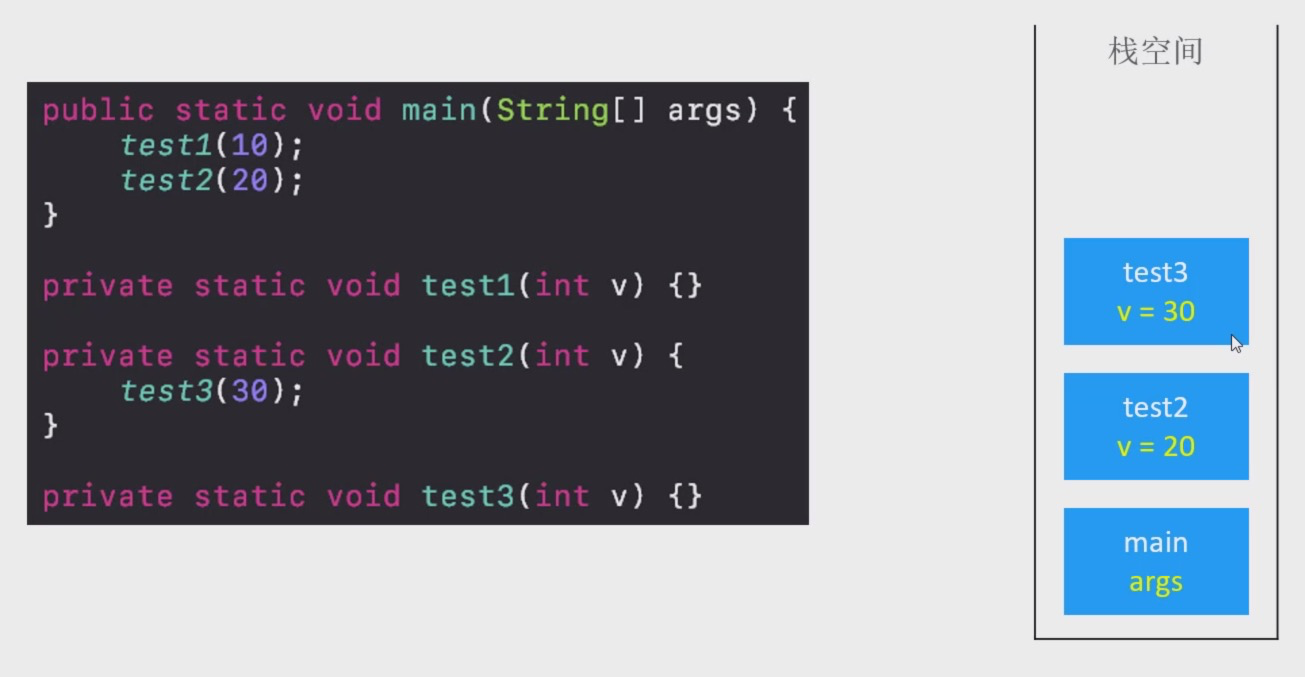## 函数的递归调用过程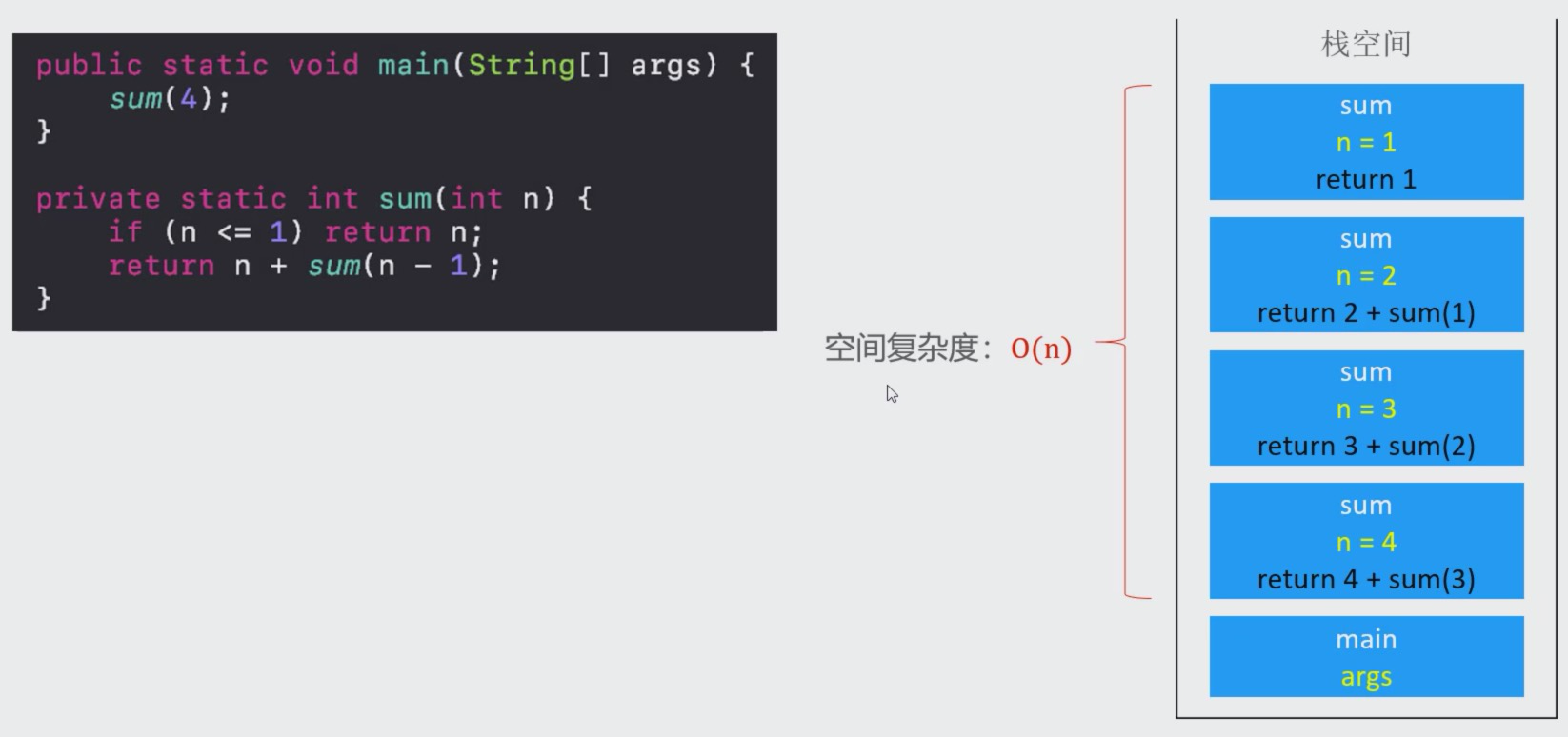• 如果递归调用没有终止，将会一直消耗栈空间
最终导致栈内存溢出（Stack Overflow）
• 所有必需要一个明确的结束递归的条件
也叫作边界条件、递归基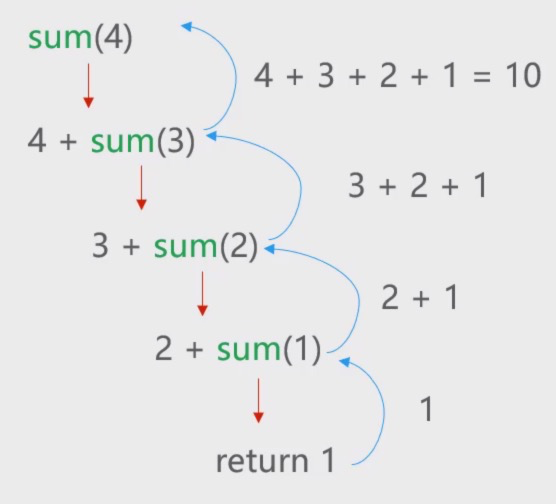## 实例分析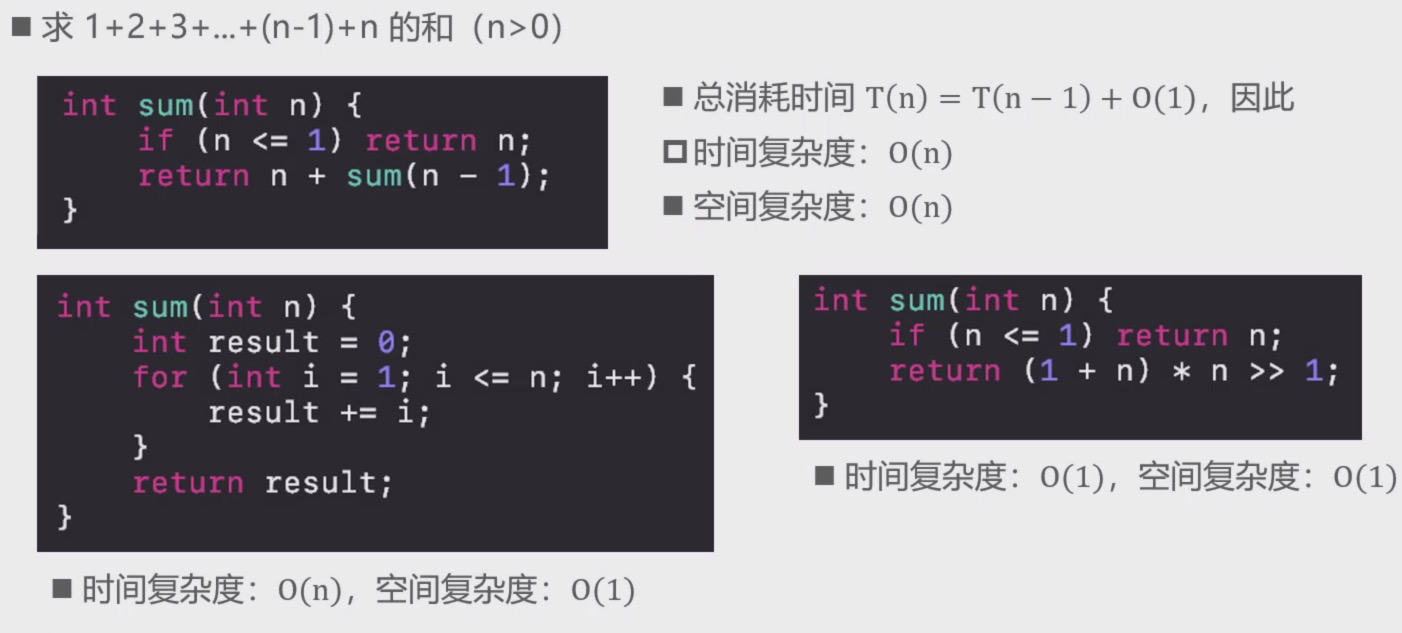• 注意：使用递归不是为了求得最优解，是为了简化解决问题的思路，代码会更加简洁
• 递归求出来的很有可能不是最优解，也可能是最优解

## 递归的基本思想

• 拆解问题
1. 把规模大的问题编程规模较小的同类型问题
2. 规模较小的问题又不断变成规模更小的问题
3. 规模小到一定程度可以直接得出它的解
• 求解
1. 由最小规模问题的解得出较大规模问题的解
2. 由较大规模问题的解不断得出规模更大问题的解
3. 最后得出原来问题的解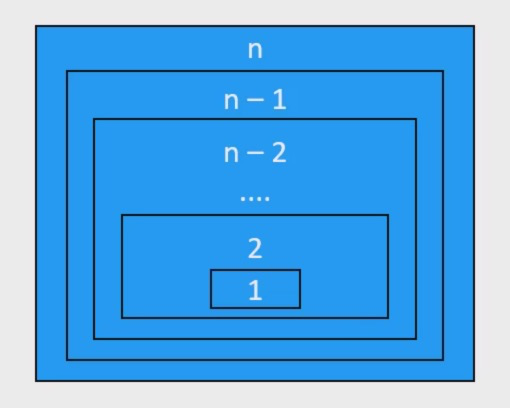1. 凡是可以利用上述思想解决问题的，都可以尝试使用递归
2. 很多链表、二叉树相关的问题都可以使用递归来解决
3. 因为链表、二叉树本身就是递归的结构（链表中包含链表，二叉树中包含二叉树）

## 递归的使用套路

1. 明确函数的功能
先不要去是靠里面代码怎么写，首先搞清楚这个函数干嘛用的，能完成什么功能？
2. 明确原问题与子问题的关系
寻找f(n)与f(n-1)的关系
3. 明确递归基（边界条件）
递归的过程中，子问题的规模在不断减小，当小到一定程度时可以直接得出它的解
寻找递归基，相当于是思考：问题规模小到什么程度可以直接得出解？

## 递归转非递归

• 递归调用的过程中，会将每一次调用的参数、局部变量都保存在了对应的栈帧（Stack Frame）中
``````public static void main(String[] args) {

log(4);
}
static void log(int n) {

if (n < 1) return;
log(n - 1);
int v = n + 10;
System.out.printIn(v);
}
``````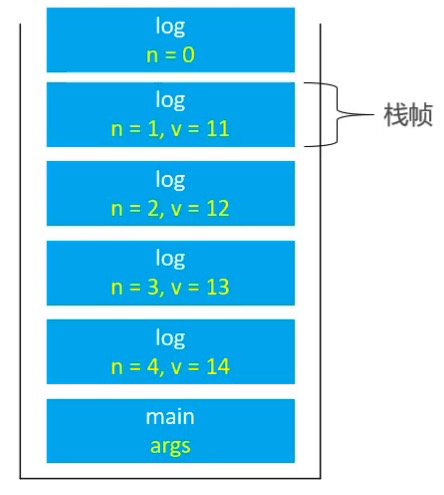• 若递归调用深度较大，会占用比较多的栈空间，甚至会导致栈溢出
• 在有些时候，递归会存在大量的重复计算，性能非常差
这时可以考虑将递归转为非递归（递归100%可以转换成非递归）
• 自己维护一个栈，来保护参数、局部变量
• 但是空间复杂度依然没有得到优化
``````static class Frame {

int n ;
int v;
Frame(int n, int v) {

this.n = n;
this.v = v;
}
}
``````
``````static void log(int n) {

Stack<Frame> frames = new Stack<>();
while (n > 0) {

frames.push(new Frame(n, n + 10));
n--;
}
while (!frames.isEmpty()) {

Frame frame = freames.pop();
System.out.printIn(frame.v);
}
}
``````
• 在某些时候，也可以重复使用一组相同的变量来保护每个栈帧的内容
``````static void log(int n) {

for (int i = 1; i <= n; i++) {

System.out.printIn(i + 10);
}
}
``````
• 这里重复使用变量i保存原来栈帧中的参数
• 空间复杂度从O(n)降到了O(1)

## 尾调用（Tail Call）

• 尾调用：一个函数的最后一个动作是调用函数
• 如果最后一个动作是调用自身，称为尾递归（Tail Recursion），是尾调用的特殊情况
``````void test1() {

int a = 10;
int b = a + 20;
test2(b);
}
``````
``````void test2(int n) {

if (n < 0) return;
test2(n - 1);
}
``````
• 一些编译器能对尾调用进行优化，以达到节省栈空间的目的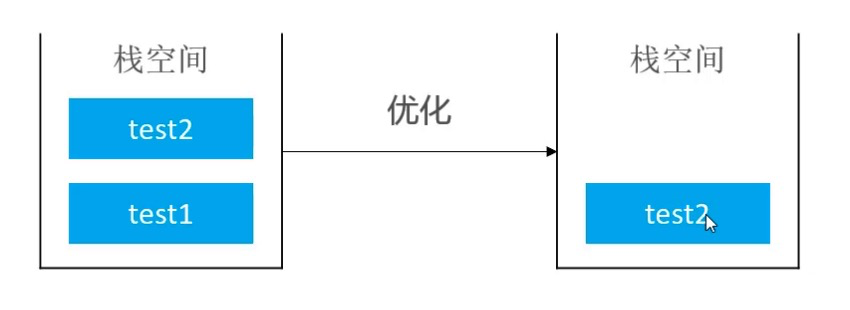• 下面代码不是尾调用
``````int factorial(int n) {

if (n <= 1) return n;
return n * factorial(n - 1);
}
``````
• 因为它最后1个动作是乘法

### 尾调用优化（Tail Call Optimization）

• 尾调用优化也叫做尾调用消除（Tail Call Elimination）
1. 如果当前栈帧上的局部变量等内容都不需要用了，当前栈帧经过适当的改变后可以直接当作被尾调用的函数的栈帧使用，然后程序可以jump到被尾调用的函数代码
2. 生成栈帧改变代码与jump的过程称作尾调用消除或尾调用优化
3. 尾调用优化让位于尾位置的函数调用跟goto语句性能一样高
• 消除尾递归里的尾调用比消除一般的尾调用容易很多
1. 比如Java虚拟机（JVM）会消除尾递归里的尾调用，但不会消除一般的尾调用（因为改变不了栈帧）
2. 因此尾递归优化相对比较普遍，平时的递归代码可以考虑尽量使用尾递归的形式

### 尾调用优化前的汇编代码（C++）

``````void test(int n) {

if (n < 0) return;
printf("test - %d\n", n);
test(n - 1);
}
``````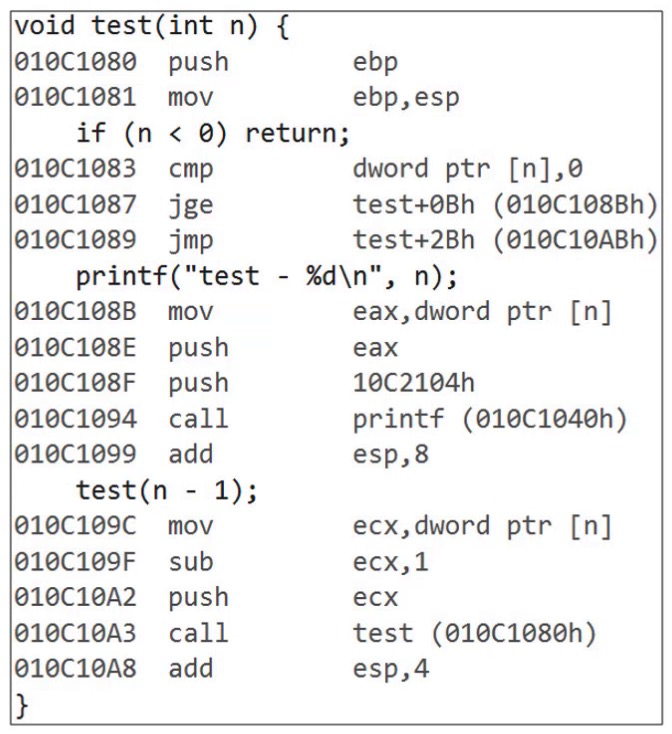### 尾调用优化后的汇编代码（C++）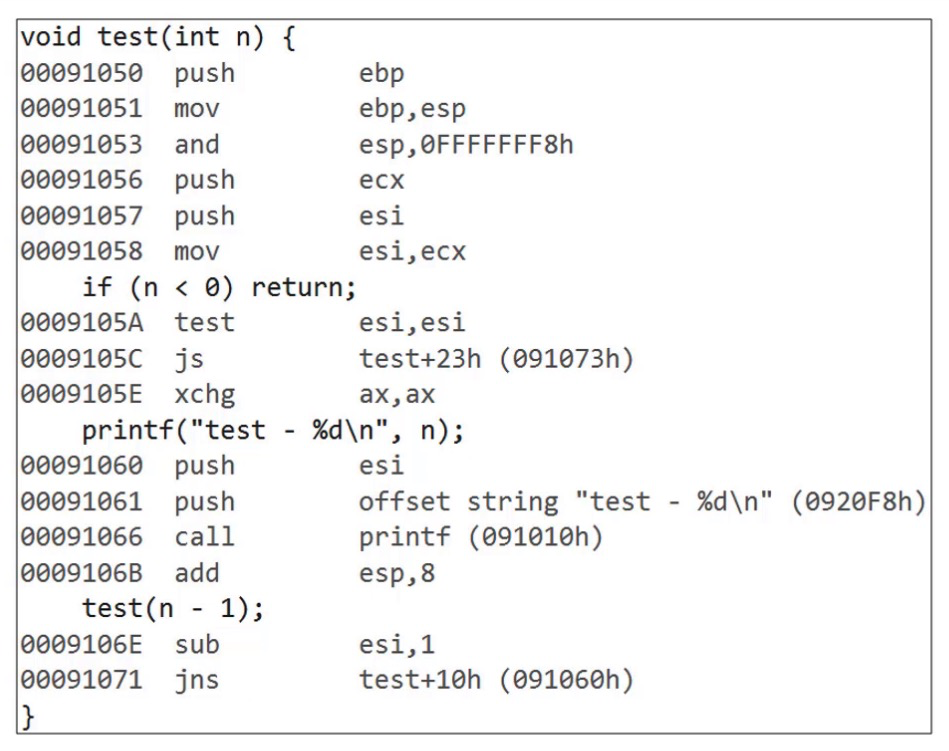### 尾递归示例1 - 阶乘

• 求n的阶乘1*2*3* … *(n - 1)*n (n>0)
``````int factorial(int n) {

if (n <= 1) return n;
return n * factorial(n - 1);
}
``````
``````int factorial(int n) {

return factorial(n, 1);
}
int factorial(int n int result) {

if (n <= 1) return result;
return factorial(n - 1, n * result);
}
``````

### 尾递归示例2 - 斐波那契额数列

``````int fib(int n) {

if (n <= 2) return 1;
return fib(n - 1) + fib(n - 2);
}
``````
``````int fib(int n) {

return fib(n, 1, 1);
}
public int fib(int n, int first, int second) {

if(n <= 1) return first;
return fib(n - 1, second, first + second);
}
``````

### linux用rdate命令实现同步时间_skate的博客-程序员宅基地

author:skatetime:2010-05-07 用rdate命令实现同步时间 前两天说到用ntp时间服务器和ntpdate命令同步时间，今天简单记录下用rdate同步时间http://blog.csdn.net/wyzxg/archive/2010/05/06/5561548.aspx   在各种linux中都有rdate命令 1. 选在

### mac 安装pyenv(python 多版本控制工具)_砚子丫的博客-程序员宅基地

git clone https://github.com/yyuu/pyenv.git ~/.pyenv配置环境变量# 编辑环境变量文件，没有就新建vim ~/.bash_profile# 配置文件内容export PATH="/Users/v_liangshuyan/.pyenv/bin:\$PATH"if which pyenv &gt; /dev/null; then eval "\$(pyenv init -)";fi# 使环境变量生效source ~/.bash_profi.

### python---get/post请求下载指定URL返回的网页内容，出现gzip乱码处理。设置Accept-Encoding为gzip,deflate，返回的网页是乱码_徐为波的博客-程序员宅基地

python—get/post请求下载指定URL返回的网页内容，出现gzip乱码处理。设置Accept-Encoding为gzip,deflate，返回的网页是乱码1、脚本# --*-- coding:utf-8 --*--#coding:utf-8import stringimport urllibimport urllib2import ssldef getpicyan